Latest Banking jobs   »

# Quantitative Aptitude Quiz For RBI Assistant/ ESIC UDC Mains 2022- 18th April

Q1. A invested 50% more than B and the ratio of time period for which A & B invested is 5: 4 respectively. If profit earned by A is Rs.840 more than the profit earned by B, then find the total profit earned by B and A both?
(a) Rs.2620
(b) Rs.2760
(c) Rs.2880
(d) Rs.2780
(e) Rs.2460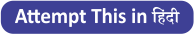Q2. Tap P can fill a tank in 24 minutes and tap Q can fill the same tank in 30 minutes, another tap R can empty the tank in 20 minutes. If tap P and tap Q are opened together & after six minutes tap R is also opened, then find the total time taken to fill the remaining tank.
(a) 18 minutes
(b) 14 minutes
(c) 22 minutes
(d)12 minutes
(e) 16 minutes

Q3. The age of A is 25% less than that of B. The age of B is 24 years more than the average age of his two sons whose total age is 40 years. Find the difference of age of A and B.
(a) 14 years
(b) 6 years
(c) 12 years
(d) 8 years
(e) 11 years

Q4. The radius of a cylinder & a sphere is same, and ratio of height and radius of cylinder is 2: 1.If the volume of sphere is 288 π cm³ then find the volume of cylinder? (in cm³)
(a) 438 π
(b) 426 π
(c) 420 π
(d) 432 π
(e) 444 π

Q5. ‘P’ women can complete a work in 4 days. ‘(P – 2)’ boys can complete the same work in 8 days, while ratio of efficiency of woman to boy is 3: 2. If ‘ P/2 ‘women &’ (P – 2)’ boys started the work & worked for only 2 days then, how many boys should replace the women to complete the remaining work in three days?
(a) 2
(b) 8
(c) 6
(d) 4
(e) 16

Q6. Ratio of difference between compound interest & simple interest for 2nd year on Rs ‘X’ at 20% p.a. compounding annually to simple interest earned for 3rd year only on Rs ‘X + 5000’ at 10% p.a. is 1 : 5. Find simple interest earned for 4 years at 15% on Rs ‘X + 10000’ is how much more than compound interest earned on Rs ‘X + 8000’ for 2 years at 15% rate only compounding annually?
(a) Rs 4654.5
(b) Rs 4468.5
(c) Rs 4192.5
(d) Rs 4807.5
(e) Rs 4324.5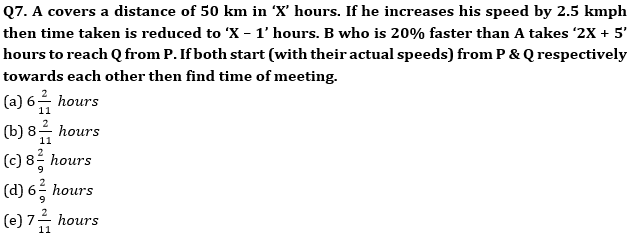Directions (8 – 9): Read the following information carefully & answer the questions.
A work can be completed by 5 members of a group A in 7 days. While another group B of 6 members can complete the same work in 6 days. Each group has at least 2 men & 2 women. Efficiency of a woman is 25% less than that of a man.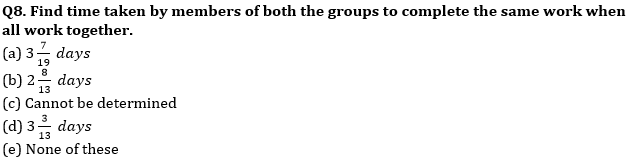Q9. If group A start working but after 3 days, they were dropped then group B was employed. After another 2 days, 2 members of the group left. Find minimum time taken to finish the whole work.
(a) 7 days
(b) 7.3 days
(c) None of these
(d) Cannot be determined
(e) 7.1 days

Q10. Three persons X, Y and Z started a business by investing in the ratio of ⅔ : ½ : ¾ . After 5 months, Y increases his investment by ⅔rd of his initial amount. If after 12 months, the difference of the profit shares of X and Z is Rs.1,350 then find the profit share of Y.
(a) Rs. 11,500
(b) Rs. 11,200
(c) Rs. 12,250
(d) Rs. 11,250
(e) Rs. 10,250

Solutions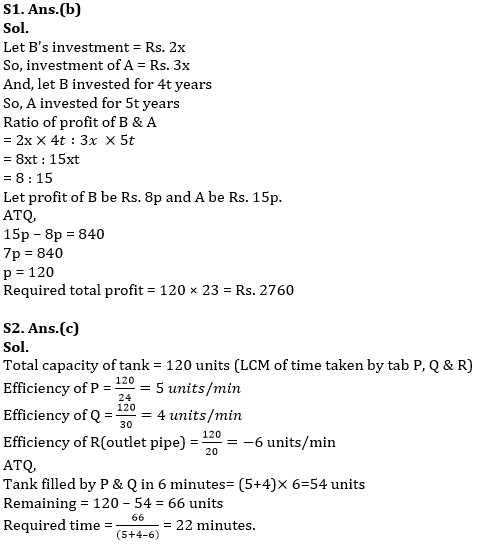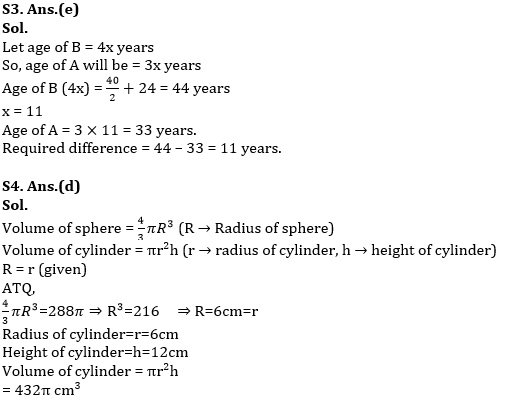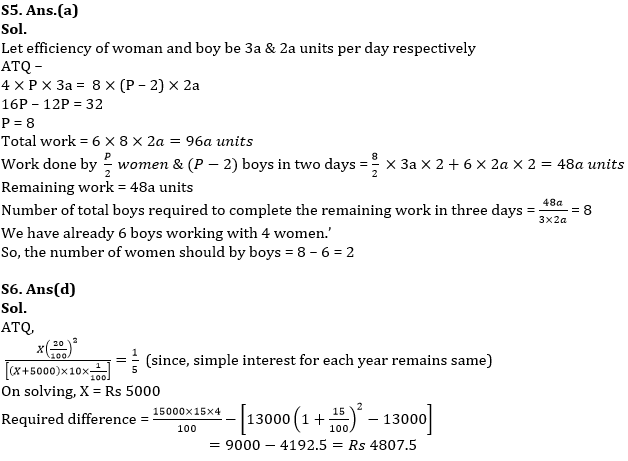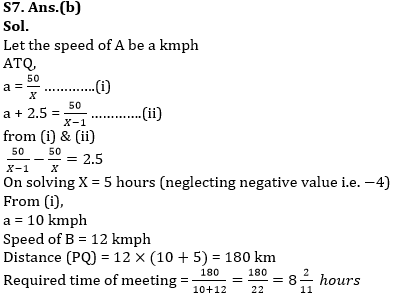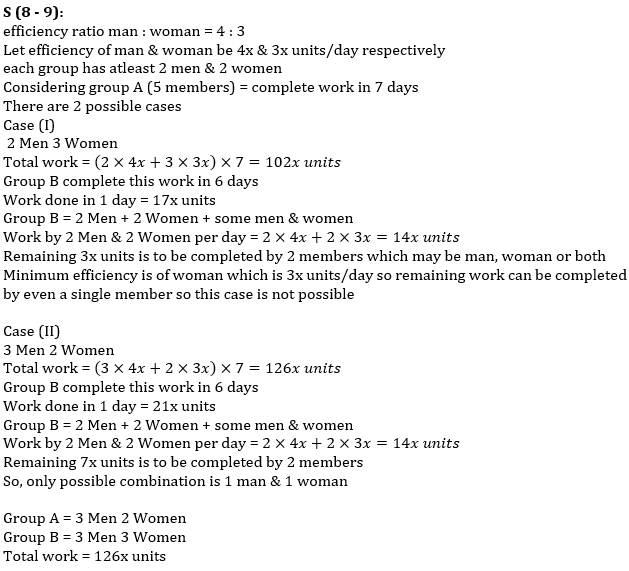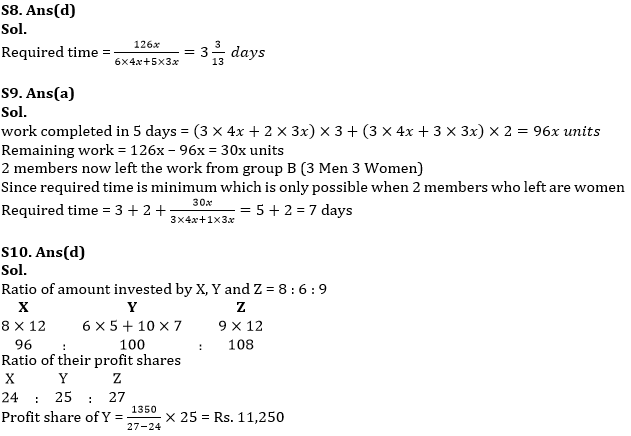#### Congratulations!Download Hindu Review of October 2021: Free PDF